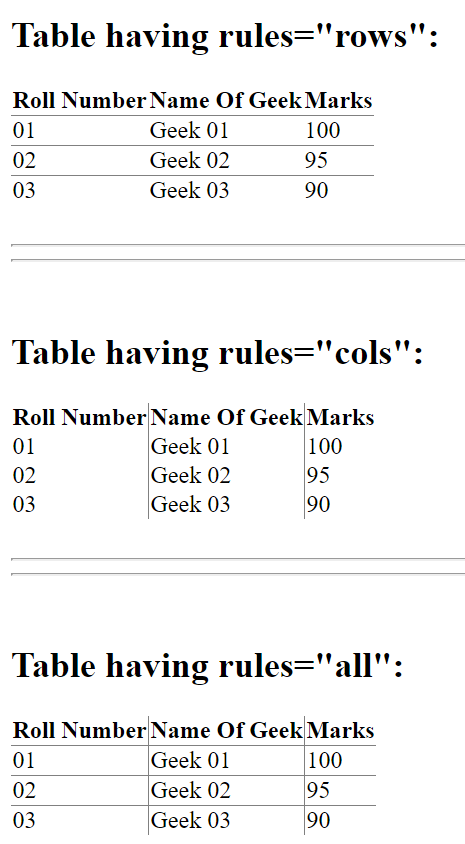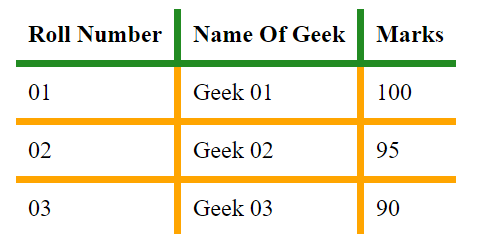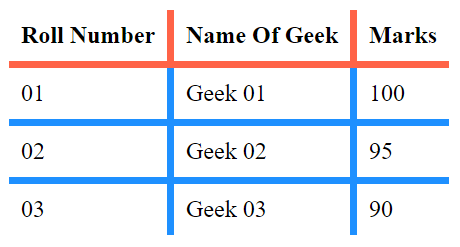# How to apply border inside a table ?

There are two ways to apply border inside the table in HTML.

• Only using HTML
• Using HTML and CSS

Only Using HTML: In this case, we will use rules attribute of table. Rules is the attribute in HTML table which allows user to display only inside borders of the table which can be choosen among only rows, cols or all.

Example:

 `` ` ` `<``html``> ` `<``head``> ` `    ``<``title``>Table In HTML ` ` ` ` `  `<``body``> ` `    ``<``h2``>Table having rules="rows": ` `    ``<``table` `rules``=``"rows"``> ` `         `  `        ``` `        ``<``tr``> ` `            ``<``th``>Roll Number ` `            ``<``th``>Name Of Geek ` `            ``<``th``>Marks ` `        `` ` `        ``<``tr``> ` `            ``<``td``>01 ` `            ``<``td``>Geek 01 ` `            ``<``td``>100 ` `        `` ` `        ``<``tr``> ` `            ``<``td``>02 ` `            ``<``td``>Geek 02 ` `            ``<``td``>95 ` `        `` ` `        ``<``tr``> ` `            ``<``td``>03 ` `            ``<``td``>Geek 03 ` `            ``<``td``>90 ` `        `` ` `    `` ` `     `  `    ``<``br``> ` `    ``<``hr``><``hr``> ` `    ``<``br``> ` `     `  `    ``<``h2``>Table having rules="cols": ` `     `  `    ``` `    ``<``table` `rules``=``"cols"``> ` `        ``<``tr``> ` `            ``<``th``>Roll Number ` `            ``<``th``>Name Of Geek ` `            ``<``th``>Marks ` `        `` ` `        ``<``tr``> ` `            ``<``td``>01 ` `            ``<``td``>Geek 01 ` `            ``<``td``>100 ` `        `` ` `        ``<``tr``> ` `            ``<``td``>02 ` `            ``<``td``>Geek 02 ` `            ``<``td``>95 ` `        `` ` `        ``<``tr``> ` `            ``<``td``>03 ` `            ``<``td``>Geek 03 ` `            ``<``td``>90 ` `        `` ` `    `` ` `     `  `    ``<``br``> ` `    ``<``hr``><``hr``> ` `    ``<``br``> ` `     `  `    ``<``h2``>Table having rules="all": ` `     `  `    ``` `    ``<``table` `rules``=``"all"``> ` `        ``<``tr``> ` `            ``<``th``>Roll Number ` `            ``<``th``>Name Of Geek ` `            ``<``th``>Marks ` `        `` ` `        ``<``tr``> ` `            ``<``td``>01 ` `            ``<``td``>Geek 01 ` `            ``<``td``>100 ` `        `` ` `        ``<``tr``> ` `            ``<``td``>02 ` `            ``<``td``>Geek 02 ` `            ``<``td``>95 ` `        `` ` `        ``<``tr``> ` `            ``<``td``>03 ` `            ``<``td``>Geek 03 ` `            ``<``td``>90 ` `        `` ` `    `` ` ` ` ` `  ` `

Output:Using HTML and CSS:

• Example 1: The border-style in CSS is another way of displaying borders inside table. This property helps user to manipulate the outside borders of the table.

 `` ` ` `<``html``> ` `     `  `<``head``> ` `    ``<``title``>Table In HTML ` `     `  `    ``<``style` `media``=``"screen"``> ` `        ``table { ` `            ``margin: 0 auto; ` `            ``border-collapse: collapse; ` `            ``border-style: hidden; ` `            ``/*Remove all the outside ` `            ``borders of the existing table*/ ` `        ``} ` `        ``table td { ` `            ``padding: 0.5rem; ` `            ``border: 5px solid orange; ` `        ``} ` `        ``table th { ` `            ``padding: 0.5rem; ` `            ``border: 5px solid ForestGreen; ` `        ``} ` `    `` ` ` ` ` `  `<``body``> ` `    ``<``table``> ` `        ``<``tr``> ` `            ``<``th``>Roll Number ` `            ``<``th``>Name Of Geek ` `            ``<``th``>Marks ` `        `` ` `        ``<``tr``> ` `            ``<``td``>01 ` `            ``<``td``>Geek 01 ` `            ``<``td``>100 ` `        `` ` `        ``<``tr``> ` `            ``<``td``>02 ` `            ``<``td``>Geek 02 ` `            ``<``td``>95 ` `        `` ` `        ``<``tr``> ` `            ``<``td``>03 ` `            ``<``td``>Geek 03 ` `            ``<``td``>90 ` `        `` ` `    `` ` ` ` ` `  ` `

Output:• Example 2: Using child concept in CSS is another way of getting a table with the inside border is by removing all the unwanted borders in the table. It can be achieved by using first-child and last-child in CSS. Here, we select the first column and remove its left-hand side border, then select the first row and remove its top border, then we go for the last column and remove its right-hand side border and at last select the last row and remove its bottom border. In this way, we remove all the outside borders of the table and we are left with inside one.

 ` ` `<``html``> ` ` `  `<``head``> ` `    ``<``title``>Table In HTML ` ` `  `    ``<``style` `media``=``"screen"``> ` `        ``table { ` `            ``margin: 0 auto; ` `            ``border-collapse: collapse; ` `        ``} ` `        ``table td { ` `            ``padding: 0.5rem; ` `            ``border: 5px solid DodgerBlue; ` `        ``} ` `        ``table th { ` `            ``padding: 0.5rem; ` `            ``border: 5px solid Tomato; ` `        ``} ` `         `  `        ``/* Removing all unwanted border ` `        ``from left hand side by calling ` `        ``all the elements in the first ` `        ``column and removing their left ` `        ``border*/ ` `        ``table tr td:first-child, th:first-child{ ` `            ``border-left: none; ` `        ``} ` `         `  `        ``/* Removing all unwanted border ` `        ``from top of the table by calling ` `        ``all the elements in first row and ` `        ``removing their top border*/ ` `        ``table tr:first-child th{ ` `            ``border-top: none; ` `        ``} ` `         `  `        ``/* Removing all unwanted border ` `        ``from right hand side by calling ` `        ``all the elements in last row and ` `        ``removing their right border*/ ` `        ``table tr td:last-child, th:last-child{ ` `            ``border-right: none; ` `        ``} ` `         `  `        ``/* Removing all unwanted border ` `        ``from bottom of the table by ` `        ``calling all the elements in ` `        ``last column and removing their ` `        ``bottom border*/ ` `        ``table tr:last-child td{ ` `            ``border-bottom: none; ` `        ``} ` `    `` ` ` ` ` `  `<``body``> ` `    ``<``table``> ` `        ``<``tr``> ` `            ``<``th``>Roll Number ` `            ``<``th``>Name Of Geek ` `            ``<``th``>Marks ` `        `` ` `        ``<``tr``> ` `            ``<``td``>01 ` `            ``<``td``>Geek 01 ` `            ``<``td``>100 ` `        `` ` `        ``<``tr``> ` `            ``<``td``>02 ` `            ``<``td``>Geek 02 ` `            ``<``td``>95 ` `        `` ` `        ``<``tr``> ` `            ``<``td``>03 ` `            ``<``td``>Geek 03 ` `            ``<``td``>90 ` `        `` ` `    `` ` ` ` ` `  ` `

Output:My Personal Notes arrow_drop_upCheck out this Author's contributed articles.

If you like GeeksforGeeks and would like to contribute, you can also write an article using contribute.geeksforgeeks.org or mail your article to contribute@geeksforgeeks.org. See your article appearing on the GeeksforGeeks main page and help other Geeks.

Please Improve this article if you find anything incorrect by clicking on the "Improve Article" button below.